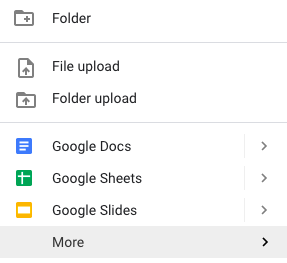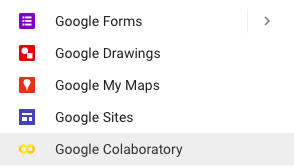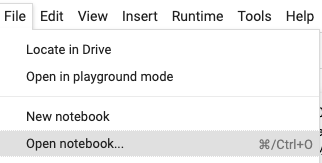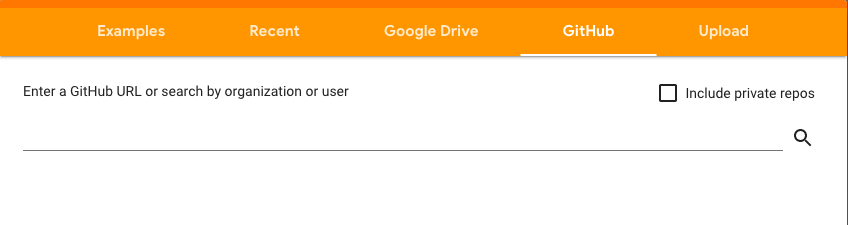# Build your first neural network in less than 1 minute (on Google Colab)

## Neural networks and why they are awesome

The brain sure as hell doesn’t work by somebody programming in rules.

–Geoffrey Hinton

In the last five years, neural networks have gone from a niche application known to only a few to a widely applied technology across many industries. Despite their popularity and success, their math makes them quite intimidating for new learners. However, the availability of many powerful and easy to learn ML libraries like TensorFlow, Keras, Pytorch, etc., has enabled us to quickly build neural network models without having to deal with the mathematics behind them. Moreover, their ability to handle various data types from multiple domains also makes learning quite fun as you can try building your neural network with data of your choice.

## Google Colaboratory and neural network code

Neural networks are resource-hungry algorithms. Simple models can be trained using CPUs on a laptop, but real-world models are usually trained using hardware accelerators like GPUs and TPUs. Colab is a free interactive development environment based on Jupyter Notebook through which you can access a GPU or a TPU and 12 GB of RAM freely for up to 12 consecutive hours. It can be installed in your Google Drive as a G Suite app. In this article we’ll see how to access Colab and how to get started with your first neural network.

You can find the code that goes with this article and open it as a Colab notebook here.

From Drive you can also open a new notebook by clicking on “+New”.

``
``

By default, no hardware is selected. You can go to the “Edit” menu of your notebook and select the hardware from the “Notebook settings” menu. Both GPU and TPU are available.

If you have an existing notebook, you can upload it to your Google Drive and open it with Google Colaboratory. You can also directly open a notebook from a Github repository by choosing the appropriate tab from the File->Open notebook… menu:## The code

Now let’s build our first neural network. But before that, we will first create some dummy data to train it. Let’s import a few packages for that:

• NumPy: a scientific computing package with classes and methods for linear algebra and N-dimensional arrays.
• matplotlib: the standard Python package for 2D plots like line and scatter plots, histograms, bar charts, etc.
• We will also import `make_circles` from sklearn, the most popular Python machine learning package.

Finally, let’s use the IPython magic function `%matplotlib inline` in order to display the generated plot directly below the corresponding Jupyter cell:

``````import numpy as np
import matplotlib.pyplot as plt
%matplotlib inline
from sklearn.datasets.samples_generator import make_circles``````

Now, let’s generate 1000 data points with two classes using `make_circles`. This function creates sample data in the form of 2D coordinate points divided into two categories: some points forming a large circle and some more forming a smaller circle. We will also introduce some noise into our data so that it doesn’t become too easy for our model to learn. The function returns two outputs, `X` and `y`, where `X` is the collection of the coordinates and `y` is the array of corresponding classes (i.e. whether each point belongs to the large or small circle).

``````X, y = make_circles(n_samples=1000,
noise=0.1,
factor=0.2,
random_state=0)``````

X is a NumPy array, so if we check its shape we can observe that it has 1000 data points with 2 coordinates:

``````array([[ 0.24265541,  0.0383196 ],
[ 0.04433036, -0.05667334],
[-0.78677748, -0.75718576],
...,
[ 0.0161236 , -0.00548034],
[ 0.20624715,  0.09769677],
[-0.19186631,  0.08916672]])``````

i.e. its shape is: (1000, 2).

We can visualize our data using Matplotlib. For that, we can use the `plot` function. Here we are representing class 0 with blue dots and class 1 with red crosses:

Now let’s build our first neural network. There are various types of neural networks, and today we will be using the simplest, which is a fully connected network. Our network will have two inputs and one hidden layer and one output layer (not sure how to size the inputs and the outputs of your network? Take a look at this other article for an in-depth explanation). We will have four neurons in our hidden layer and one neuron in the output layer.

To build the network, we will be using the Keras API from TensorFlow 2. We will import the `Sequential` class, the `Dense` layer, and the Stochastic Gradient Descent optimizer (`SGD`).

``````from tensorflow.keras.models import Sequential
from tensorflow.keras.layers import Dense
from tensorflow.keras.optimizers import SGD``````

The `Sequential` model class allows you to define deep learning models by stacking up layers in a list. Here, we will create a `Sequential` object with two dense layers. One dense layer is the hidden layer we mentioned above, and the next dense layer is the output layer. The `tanh` activation used in the first layer enables the model to create a non-linear decision boundary. This is essential as the decision boundary for our dataset should be approximately circular. We are using the `sigmoid` activation in our output layer. This activation limits our output to a range between 0 and 1, which can be treated as the probability of belonging to the class labeled as 1 (i.e. the red crosses in our case).

``````model = Sequential([
Dense(4, input_shape=(2,), activation='tanh'),
Dense(1, activation='sigmoid'),
])``````

In Keras, after building a model, we need to compile it. By compiling the model, we configure the learning process of our network. We will be using binary cross entropy as our loss function, which checks how well our model is performing by comparing its predictions with the input labels. While training the network, our goal is to reduce this loss. We achieve this by using Stochastic Gradient Descent (`SGD`) with a learning rate of 0.5. We will also monitor the model performance through the accuracy score.

``````model.compile(SGD(lr=0.5),
'binary_crossentropy',
metrics=['accuracy'])``````

Once we have compiled the model, we can train it by calling the `model.fit` method. We will set the epochs to 15, where epochs indicate the number of times training will be iterated using the entire training dataset. We have to iterate the training for multiple epochs as our network might not perform optimally in the first epoch. We can see this happening in our training. Here, the loss was high in the first epoch, but it gradually decreases. At the same time, our accuracy has improved from 63% to 100%.

``model.fit(X, y, epochs=15)``
``````Epoch 1/15
1000/1000 [======] - 0s 476us/sample - loss: 0.6695 - accuracy: 0.6390
Epoch 2/15
1000/1000 [======] - 0s 204us/sample - loss: 0.5831 - accuracy: 0.7710
Epoch 3/15
1000/1000 [======] - 0s 142us/sample - loss: 0.4596 - accuracy: 0.9460
Epoch 4/15
1000/1000 [======] - 0s 119us/sample - loss: 0.3367 - accuracy: 0.9970
Epoch 5/15
1000/1000 [======] - 0s 89us/sample - loss: 0.2437 - accuracy: 1.0000
…``````

After completing the training, you can check how our model makes decisions to classify our data. Use the `contourf` function from `matplotlib` to draw the contours of our model’s decision boundary and overlay that onto our training data plot. We can observe that our model has created a somewhat triangular decision boundary to separate out these two classes:

## Going beyond the basics

Congratulations for successfully training your first neural network. This was your first step into the world of neural networks, and there are many more to go. For starters, you can tinker with the model you build. Why not change the number of neurons in your first layer and see how differently your model performs? Or add one extra dense layer and see if the shape of the decision boundary changes. You will get some insights into how neural networks behave by trying out these activities. You can also dive deeper into neural networks by taking a course called Zero to Deep Learning. Sign up here to get started.Previous Article

### How to troubleshoot shape mismatch errors in Keras/TensorFlowNext Article